# Mensuration(2D & 3D)

## Introduction

Mensuration is a division of mathematics in which we study geometric figure calculation and its parameters such as area, length, surface area, etc.

Mensuration in 2D majorly deals with finding the area and perimeter of 2D objects. In the case of the 3D objects, it majorly deals with finding the volume and surface area of 3D objects.

## 2D Mensuration

In 2D Mensuration, we will deal with the objects like triangles, squares, rectangles, circles, parallelograms and determine the area and parameter.

Let us discuss formulas for  some 2D objects:

### Triangle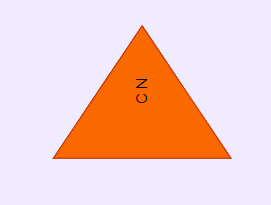Let us assume the side of the Triangle.

Note :S=(a+b+c)/2

### Rectangle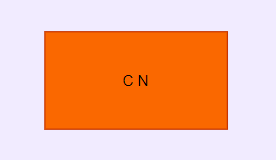•

Let us assume the side of the rectangle to be

### Square

•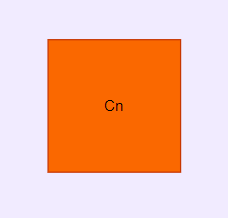Let us assume the side of the square:

### Parallelogram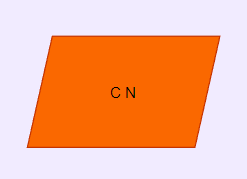### Rhombus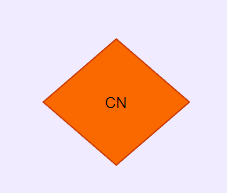### Trapezium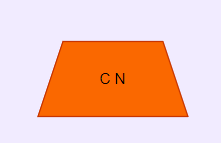### Circle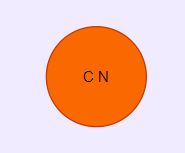## 3D Mensuration

In 3D Mensuration, we will deal with the objects like cubes, cuboids, Sphere etc. we will also determine the volume and surface area.

• Volume is a measure of the capacity that an object can hold.

• Surface area is the total area that the surface of an object occupies.

Let us discuss some 3D objects:

### Cube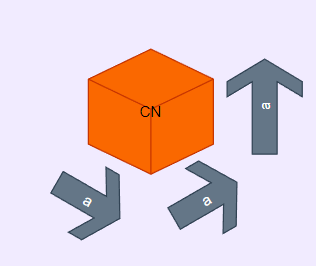Let us assume the side of a cube as mentioned in the following figure.

### Cuboid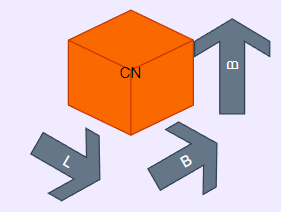Let us assume

### Cone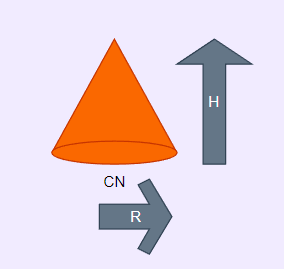Let us assume

### Cylinder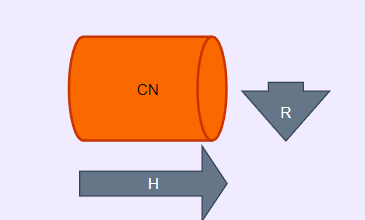Let us assume

### Hollow Cylinder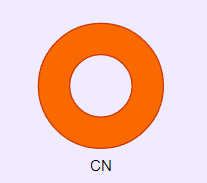Let us assume

### Sphere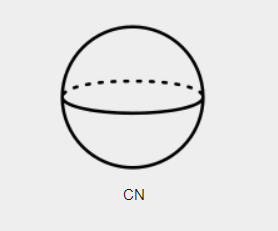Let us assume

### Hemisphere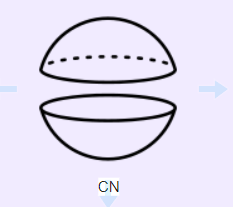Let us assume

## Problems on Mensuration

Let us discuss some problems on Mensuration:

1. A circle whose radius given to us is 21 cm, then Find its Circumference and area. (Use π = 22/7).

Solution: Since we know that,

Circumference of circle is given by = 2πr = 2 x (22/7) x 21 = 2 x 22 x 3 = 132 cm

Area of circle is give by = πr2 = (22/7) x 212 = 22/7 x 21 x 21 = 22 x 3 x 21

Thus, the area of a circle with a radius, 21cm = 1386 cm2

2. If the side of a square is given by  4 cm, what will be the area and perimeter square?

Solution: Given,

The length of the side of square given to us is = 4 cm

Than the area is given by = side2 = 42= 4 x 4 = 16 cm2

We know that perimeter of square = sum of all its sides

Since, all the sides of the square are equal,

therefore:Perimeter = 4+4+4+4 = 16 cm.

3. Suppose a quadrilateral is given to us having a diagonal of length 10 cm, which divides the quadrilateral into two triangles. The heights of triangles with diagonals as the base are given by 4 cm and 6 cm. Then, Find the area of the quadrilateral.

Solution: Given,

Diagonal of quadrilateral , d = 10 cm

Consider the height of one triangle, h1 = 4cm

Consider the height of another triangle, h2 = 6cm

Thus the area of quadrilateral = ½ d(h1+h2) = ½ x 10 x (4+6) = 5 x 10 = 50 sq.cm.

4. Given to us is a rhombus having diagonals of length 10 cm and 16 cm, respectively than Find its area.

Solution: Consider the diagonal first d1 = 10 cm

Consider the diagonal second d2 = 16 cm

Thus we know that ,area of rhombus (A) = ½ d1 d2

A = ½ x 10 x 16

A= 80 cm2

5. Consider a statement in which the area of a trapezium-shaped field given to us is 480 m2. The distance between two parallel sides is 15 m and provided one of the parallel sides is 20 m, find the other parallel side.

Solution: One of the parallel sides of the trapezium is a = 20 m. Let us assume another parallel side be b, height to be h = 15 m.

The given area of trapezium = 480 m2

Since We know, by formula;

Area of a trapezium = ½ h (a+b)

480 = ½ (15) (20+b)

20 + b = (480×2)/15

Hence ,b = 64 – 20 = 44 m

6. Consider a statement in which the height, length and width of a cuboidal box are 20 cm, 15 cm and 10 cm, respectively. Find its area.

Solution: Total surface area = 2(LB+BH+LH)

Total surface area =2 (20 × 15 + 20 × 10 + 10 × 15)

Thus ,TSA = 2 ( 300 + 200 + 150) = 1300 cm2

7. If a cube is given to us with its side length equal to 5cm, then calculate its area?

Solution: Given,

side-length (l)= 5 cm

Thus, Area = 6l2 = 6 x 5 x5 = 150 sq.cm

8. Consider a statement in which we have to Find the height of a cylinder whose radius is 7 cm and the total surface area given to us is 968 cm2.

Solution: Let us assume the  height of the cylinder = h

Given, radius ( r ) = 7cm

We know that ,Total surface area = 2πr (h + r)

TSA = 2 x (22/7) x 7 x (7+h) = 968

Hence ,h = 15 cm

9. Find the height of a cuboid whose volume is 275 cm3 and the base area is 25 cm2.

Solution: Volume of cuboid = l × b × h

Base area = l × b = 25 cm2

Hence,

275 = 25 × h

h = 275/25 = 11 cm

10. Consider a statement in which a rectangular piece of paper 11 cm × 4 cm is folded without overlapping. In such a way, we form a cylinder of height 4 cm then calculate the volume of the cylinder.

Solution: As per the question

The length of the paper will be equal to the perimeter of the base of the cylinder, and the width will be its height.

We know that the Circumference of the base of the cylinder = 2πr = 11 cm

2 x 22/7 x r = 11 cm

Thus r = 7/4 cm

Volume of cylinder = πr2h = (22/7) x (7/4)2 x 4

= 38.5 cm3

### Question 1: What is Mensuration?

Answer: Mensuration is a division of mathematics in which we study geometric figure calculation and its parameters such as area, length, surface area, etc.

### Question 2:What is the perimeter?

Answer: Perimeter is the outer boundary of the 2D figure.

### Question 3: What is Area?

Answer: It is the region covered by the 2D figure, or in simpler terms, we can say that it is the region occupied by a figure.

## Key takeaways

In this blog, we will discuss the problem of Mensuration and the approach to solving the problem on 2D and 3D

Mensuration.